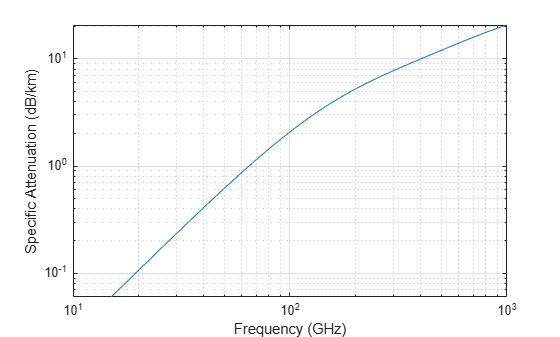Documentation

# fogpl

RF signal attenuation due to fog and clouds

## Syntax

``L = fogpl(R,freq,T,den)``

## Description

example

````L = fogpl(R,freq,T,den)` returns attenuation, `L`, when signals propagate in fog or clouds. `R` represents the signal path length. `freq` represents the signal carrier frequency, `T` is the ambient temperature, and `den` specifies the liquid water density in the fog or cloud.The `fogpl` function applies the International Telecommunication Union (ITU) cloud and fog attenuation model to calculate path loss of signals propagating through clouds and fog . Fog and clouds are the same atmospheric phenomenon, differing only by height above ground. Both environments are parameterized by their liquid water density. Other model parameters include signal frequency and temperature. This function applies when the signal path is contained entirely in a uniform fog or cloud environment. The liquid water density does not vary along the signal path. The attenuation model applies only for frequencies at 10–1000 GHz.```

## Examples

collapse all

Compute the attenuation of signals propagating through a cloud that is 1 km long at 1000 meters altitude. Compute the attenuation for frequencies from 15 to 1000 GHz. A typical value for the cloud liquid water density is 0.5 $g/{m}^{3}$. Assume the atmospheric temperature at 1000 meters is ${20}^{\circ }$C.

```R = 1000.0; freq = [15:5:1000]*1e9; T = 20.0; lwd = 0.5; L = fogpl(R,freq,T,lwd);```

Plot the specific attenuation as a function of frequency. Specific attenuation is the attenuation or loss per kilometer.

```loglog(freq/1e9,L) grid xlabel('Frequency (GHz)') ylabel('Specific Attenuation (dB/km)')```## Input Arguments

collapse all

Signal path length, specified as a scalar or as an M-by-1 or 1-by-M vector of nonnegative real-values. Total attenuation is the specific attenuation multiplied by the path length. Units are meters.

Example: `[1300.0,1400.0]`

Signal frequency, specified as a positive real-valued scalar or as an N-by-1 nonnegative real-valued vector or 1-by-N nonnegative real-valued vector. Frequencies must lie in the range 10–1000 GHz.

Example: `[14.0e9,15.0e9`]

Ambient temperature in fog or cloud, specified as a real-valued scalar. Units are in degrees Celsius.

Example: `-10.0`

Liquid water density, specified as a nonnegative real-valued scalar. Units are g/m3. Typical values for liquid water density in fog range from approximately 0.05 g/m3 for medium fog to approximately 0.5 g/m3 for thick fog. For medium fog, visibility is about 300 meters. For heavy fog, visibility is about 50 meters. Cumulus cloud liquid water density is typically 0.5 g/m3.

Example: `0.01`

## Output Arguments

collapse all

Signal attenuation, returned as a real-valued M-by-N matrix. Each matrix row represents a different path where M is the number of paths. Each column represents a different frequency where N is the number of frequencies. Units are in dB.

collapse all

### Fog and Cloud Attenuation Model

This model calculates the attenuation of signals that propagate through fog or clouds.

Fog and cloud attenuation are the same atmospheric phenomenon. The ITU model, Recommendation ITU-R P.840-6: Attenuation due to clouds and fog is used. The model computes the specific attenuation (attenuation per kilometer), of a signal as a function of liquid water density, signal frequency, and temperature. The model applies to polarized and nonpolarized fields. The formula for specific attenuation at each frequency is

`${\gamma }_{c}={K}_{l}\left(f\right)M,$`

where M is the liquid water density in gm/m3. The quantity Kl(f) is the specific attenuation coefficient and depends on frequency. The cloud and fog attenuation model is valid for frequencies 10–1000 GHz. Units for the specific attenuation coefficient are (dB/km)/(g/m3).

To compute the total attenuation for narrowband signals along a path, the function multiplies the specific attenuation by the path length R. Total attenuation is Lc = Rγc.

You can apply the attenuation model to wideband signals. First, divide the wideband signal into frequency subbands, and apply narrowband attenuation to each subband. Then, sum all attenuated subband signals into the total attenuated signal.

 Radiocommunication Sector of International Telecommunication Union. Recommendation ITU-R P.840-6: Attenuation due to clouds and fog. 2013.